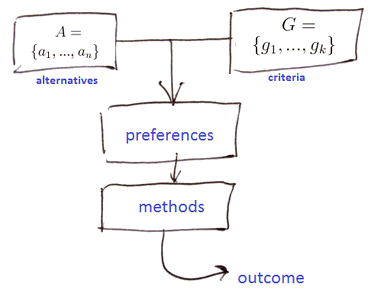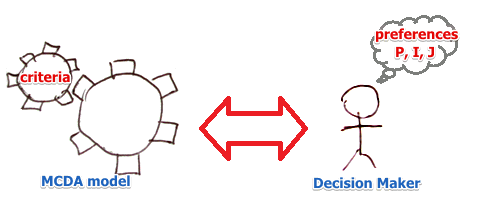# ML Wiki

## Multi-Criteria Decision Aid

This is a tool that helps a decision maker to choose a solution when he is facing conflicting criteria and cannot decide.

For example, you want to buy a new car:

• One is expensive, speed is good;
• another is cheap but slow and with little comfort.
• These criteria (cost vs speed) are conflicting.

We need to find a compromise that answers the expectation of a decision maker• Step 1: Define the set of alternatives $A = \{a_1, ..., a_n\}$
• Step 2: Define the set of criteria $G = \{g_1, ..., g_k\}$
• Step 3: Define the Preferences (the expectations of a decision maker)
• Step 4: Apply methods to find the best alternative

## Alternatives

$A$ - set of alternatives (actions, options, items, decisions, etc)

$A$ can be

• finite or infinite
• countable or uncountable
• stable (always the same) or evolving

## Criteria

A criterion $g_i$ is a mapping from the set of alternatives $A$ to some totally ordered set $E_i$:

• $g_i: A \mapsto E_i$
• $g_i \in G$ form a set of criteria

With $E_i$ we can rank all elements of $A$ from best to worst

Examples:

• $E = \mathbb{R}$
• $E = \{\text{VB}, \text{B}, \text{M}, \text{G}, \text{VG}\}$

A set can be:

• ordinal (operations $<, =, >$)
• $E = \{\text{VB}, \text{B}, \text{M}, \text{G}, \text{VG}\}$
• interval (operations $<, =, >, +, -$)
• temperature
• ratio (operations $<, =, >, +, -, \cdot, /$)
• $E = \mathbb{R}$

Restrictions on $G$:

### Dominance Principle

Some alternatives can be eliminated by Dominance principle

• If for two alternatives $a$ and $b$ for all criteria they are equally good
• but there exists one criteria at which $a$ is better than $b$
• then $b$ is dominated by $a$ and will never be chosen

Consider this example

• we're choosing a car
• there are 4 criteria: price, power, consumption, comfort
• there are 6 alternatives
Price Power Consumption Comfort
Avg A. 18 75 8 3
Sport 18.5 110 9 2
Avg B. 17.5 85 7 3
Lux 1 24 90 8.5 5
Exonomic 12.5 50 7.5 1
Lux 2 22.5 85 9 4

By Dominance principle:

• We see that Avg B is always better than Avg. A
• then nobody will ever choose Avg A: A is dominated by B
• but no other alternative can be eliminated this way

How to chose which one is the best?

• Need subjective preferences

## Preferences

To be able to find the best solution we need to know subjective preferences

Given two alternatives $a$ and $b$ a decision maker can say if

• $a \ P \ b$ or $b \ P \ a$: $a$ is preferred to $b$ - the preference relation
• $a \ I \ b$ - the indifference relation (not transitive! see Luce's Coffee Cups)
• $a \ J \ b$ - the incomparability relation, when you cannot compare thingsHow to represent a Decision Maker's preferences in some model?

With Modeling Preferences:

Important condition when modeling preferences:

## Methods

There some important families of criteria:

### Outranking

Outranking methods perform pair-wise comparisons (like in the Condorcet's Rule)

Most famous methods:

Problems of outranking methods:

## Multi-Objective Optimization

Once we found the Pareto-optimal set of solutions in a problem, we need to find the best solution, and MCDA can help with it